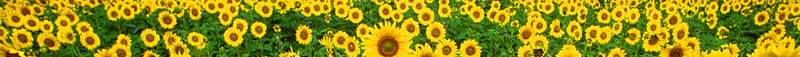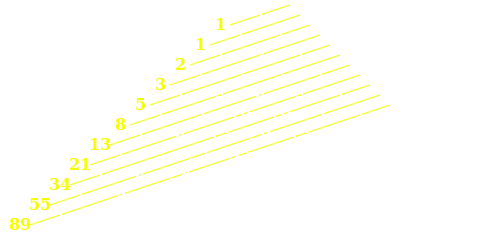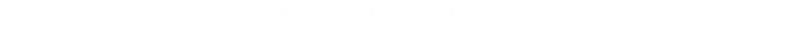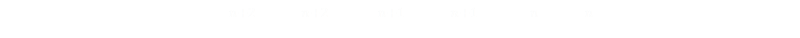﻿ Generating Fibonacci Numbers# Generating Fibonacci Numbers

Fibonacci sequences are one of the most well-known sequences in mathematics.

1, 1, 2, 3, 5, 8, 13, 21, 34, 55, 89, 144 …*

The sequence is named after Leonardo Bonacci (also known as “Fibonacci” 1170-1250), who is considered to be “the most talented Western mathematician of the Middle Ages”.

Each number in the sequence is generated by adding together the two previous numbers.

Fn = Fn-1 + Fn-2

*People argue if the sequence should start at zero or one. I’m going to define F0=1

### Everywhere

You’ll find Fibonacci numbers occur in lots of places (both in nature and mathematics), and the sequence is intimately related to the Golden Ratio φ (Even more has been written about the Golden Ratio than Fibonacci. Some of it is even true, but there is also a lot of ‘pseudo-science’, and some statements about it are just plain wrong or made up! If someone tells you some interesting ‘fact’ about the Golden Ratio, apply a common sense filter first before thinking about believing it).The ratio of two adjacent terms in the Fibonacci sequence is an approximation for the Golden Ratio φ

The larger the terms used, the better the approximation, so 1597/987 is a better approximation than 34/21.

F0 F1 F2 F3 F4 F5 F6 F7 F8 F9 F10 F11 F12 F13 F14 F15 F16 F17 F18 F19
1 1 2 3 5 8 13 21 34 55 89 144 233 377 610 987 1597 2584 4181 6765

To no surprise of anyone*, you can also find Fibonacci numbers in Pascal’s triangle.*There are simply so many fascinating facts, sequences, and properties about Pascal’s triangle such that, when someone discovers some new property, nobody seems surprised or shocked, and the only people genuinely excited and interested by the new discovery are the discoverer themselves, and the people who write books about Pascal's triangle :)

### Generating Fibonacci Numbers

Now that we known how Fibonacci numbers are defined, how would we go about generating them in code?

The first idea that might spring to mind is recursion. Because subsequent Fibonacci numbers are self similar versions of themselves we can write some pretty compact code to generate them by recursively calling the same function, only stopping when we get to the first couple of numbers. Here's some pseudo code to show the idea (I'm assuming we're only working with positive indices of Fibonacci):

Function Fibonacci(n)

If n <= 1 Then

Fibonacci = 1

Else

Fibonacci = Fibonacci(n-1) + Fibonacci(n-2)

End if

End Function

That code certainly looks very neat, and is easy to understand. It gives the correct ansswer, but it's a horrible solution.

Horrible, Horrible, Horrible    Can you see why?

To see why, let's instrument it a little. I'll put a print statement in there to show what's going on:

Function Fibonacci(n)

Print "Hello World n=";n

If n <= 1 Then

Fibonacci = 1

Else

Fibonacci = Fibonacci(n-1) + Fibonacci(n-2)

End if

End Function

Each time our function is called, we'll output a message as we pass through. Let's see some sample output for low values of n

n=0 n=1 n=2 n=3
Hello World n=0 Hello World n=1 Hello World n=2
Hello World n=1
Hello World n=0
Hello World n=3
Hello World n=2
Hello World n=1
Hello World n=0
Hello World n=1

So far so good. For n=0,1,2 the results are trivial. We can start to see the problem, however, for n=3.

To calculate the first part, Fibonacci(n-1), we have to go through 2,1,0. Then for the the second part, Fibonacci(n-2) we have to go through 1. Again, not so bad, but there are a couple of problems. The first is we're calling the same function multiple times, staggered (which should give us the same answer just phase shifted), and the second problem is we're (wastefully) recalcuating the middle part each time. Let's so how much this quickly blows up. Here it is with n=4, 5, 6:

n=4 n=5 n=6
Hello World n=4
Hello World n=3
Hello World n=2
Hello World n=1
Hello World n=0
Hello World n=1
Hello World n=2
Hello World n=1
Hello World n=0
Hello World n=5
Hello World n=4
Hello World n=3
Hello World n=2
Hello World n=1
Hello World n=0
Hello World n=1
Hello World n=2
Hello World n=1
Hello World n=0
Hello World n=3
Hello World n=2
Hello World n=1
Hello World n=0
Hello World n=1
Hello World n=6
Hello World n=5
Hello World n=4
Hello World n=3
Hello World n=2
Hello World n=1
Hello World n=0
Hello World n=1
Hello World n=2
Hello World n=1
Hello World n=0
Hello World n=3
Hello World n=2
Hello World n=1
Hello World n=0
Hello World n=1
Hello World n=4
Hello World n=3
Hello World n=1
Hello World n=1
Hello World n=0
Hello World n=1
Hello World n=2
Hello World n=1
Hello World n=0Let's take a look at what is happening. On the left is a tree of the how the function is recursively called. You can immediately see how complex things are nested and now n=1 is redundently calculates five times.

It's a horrible, horrible, Ponzi scheme of an algorithm. Each subsequent depth takes the entire previous two parts and wraps them inside it's own misery. To see how badly this escalates here is a count of the number of times 'Hello World' would be printed out if you adopted this algoriothm:

F0 F1 F2 F3 F4 F5 F6 F7 F8 F9 F14 F15 F29 F39
1 1 3 5 9 15 25 41 67 109 1,219 1,973 1,664,079 204,668,309

That's crazy, to calculate F39 we need to pass through the function over 200 million times!

There have to be better ways.### Memoization

If we could remember previous results we would not have to go back down the tree to calculate answers that we've already seen.

This is technique called memoization. Each time we need to determine a Fibonacci value we check to see if we've calculated it already; if so we use thie already precalculated value. If not, we calculate it just once and store it away for later benefit.

'setup

Function Fibonacci(n)

else

Fibonacci = Fibonacci(n-1) + Fibonacci(n-2)

End if

End Function

In this code, we pre-populate F0 and F1 upon initialization.

Then, when the function is called, we first check to see if we have this value in the cache. If so, we return it and are done.

If we have not calculated this value yet we recurse in, as before, but each call checks the to see if we come across a known value and short circuit at this point. Upon calculating this new value we add it to the cache for future benefit.

The very first time this function is called, the code has to run all the way down to the bottom, then come back up again, saving values as it comes back up. Then, if called again with the same value (or any value less), then the answer is returned immediately.

If a subsequent call is made for a Fibonacci value higher than the current highest value known, then the function only recurses down until it reaches the highest value previously seen (and we don't need to go all the way down to 0).

Let's see this in operation and, as before, we'll instrument it.

'setup

Function Fibonacci(n)

Print "Hello World n=";n

else

Fibonacci = Fibonacci(n-1) + Fibonacci(n-2)

End if

End Function

Below, on the left we see how it perfoms for n=5 on first run (nothing in cache). It runs all the way to the bottom, then comes back up again (as it comee back up, it writes the values in the cache). The second column below shows what happens if we then call the function with n=3 (a lower value), and this instantly returns the known cache value. The same happens if we call the function with n=5 again a second time (next column over).

Finally, on the far column we see what happens if we call into into the function with n=11 (after we've already calculated n=5), and you can see it runs down only until five, then back up again.

n=5
[First run] →
n=3
n=5
[Second run] →
n=11
Hello World n=5
Hello World n=4
Hello World n=3
Hello World n=2
Hello World n=1
Hello World n=0
Hello World n=1
Hello World n=2
Hello World n=3
Hello World n=3 Hello World n=5
Hello World n=11
Hello World n=10
Hello World n=9
Hello World n=8
Hello World n=7
Hello World n=6
Hello World n=5
Hello World n=6
Hello World n=7
Hello World n=8
Hello World n=9

This is massively better than the first recursive way, but can we do better? Well, yes we can. In the above implementation, we're starting at the top, but if there is nothing below to build on, when we request a new higher number, we have to recurse down until we hit a known number, then go back up again. How above we reverse this and go the other way? Instead, if we start at the last known highest value and go upwards until we hit our target, then we only have to go in one direction.

### High Water Mark Upwards

As hinted above, if we start with the last-known highest number and calculate upwards we're guaranteed to not have to back track as we're building monotonically from the answers we already have. This is the method you'd use if you were calculating values by hand!

In this implementation, we have the concept of a 'high-water mark', which is the highest known value in the cache. Below this value, it's a single look-up. Above this value, we calculate upwards from the high water mark, serializing all the intermeriadate values as we pass them on the way to the desired number.

'setup

high_water_mark = 1

Function Fibonacci(n)

else

for i = (high_water_mark + 1) to n

next

high_water_mark = n

End if

End Function

As before, we've also applied memoization so that, once we've calculated an answer once, we never need to visit again.

This code is far smarter than our first implementation.

Even without a "Hello World" debug print, we can see that the code does not call itself and the function is not recursive. It either returns immediately with a value from the cache, or loops from the last known value upwards.

### Memory vs Processing

The above code is pretty efficient. It only calculates when it needs to. A downside of this, however, is that a cache is needed. Every previously calculated value needs to be stored in memory. In this example, for Fibonacci, it's pretty trivial and the memory required to store intermediate values is very small.

More generically, however, these kind of trade-offs are what programmmers and engineers balance every day. If you are going to make use of a value many times, is it better to take the memory footprint and cache it, or throw it away and take the processing hit later if you have to recalculate it from scratch?There's no right answer. Each case depends on the circumstances, use-cases, and hardware limits. Sometimes recalculating is 'better', sometimes storing is 'better'. If you are particularly memory constrained, you might need to calculate every time. Sometimes there is assymetry in access costs (such as high latency to access previous results), or high premiums to transfer data. If you want to speed up rendering of web application, for instance, it might be better to highly compress data that is transmitted from the server to the client. Even if the decompression on the client end is gnarly and takes 1000x longer to generate than simply having the clear text, this compression might greatly speed up page rendering as client side processing is a 'cheap' resource. The additional compression will shorten the time needed for the data to be sent over the internet, and this is by far the longest part of the process (oh, and you'll also save on egress costs, and your customers will be happier, not just because your application runs faster, but you will be saving them money if they pay for data by the byte!)

For this trivial Fibonacci example, if the values needed were being used frequently, I would certainly cache them. Upon initialization, I'd pre-compute the known range of all values used and simply have them in an array ready for instant recall. However, if we can't take the memory footprint, in this example, can we do better?

## Can we do better?What would be ideal is if there were a formula for Fibonacci. If there were a function/equation into which we could pass n and it would return Fn then we could calculate just the value we needed and not need to worry about intermediate or past values. Does such a function exist? The answer is yes!

### Binet Formula

Time for a little math …

Let’s imagine we can write the Fibonacci sequence as a power series:We know from definition that each term is the sum of the previous two.If we divide the first by x4 or the second by x2, we can see we get the generic result of:We can solve this using the standard quadratic equation:There are two solutions. You may have noticed that one of these roots is φ the Golden Ratio. We’ll call the other one τNow we have two power series that both satisfy our requirement that subsequent terms are created by the sum of the previous two:This is promising. Both of them have the first term as 1 (which is what we’re looking for), and they are both "Fibonacci-esque" (with each term being the sum of the two previous) but they don’t have 1 for their second terms. So, let’s do something clever. Any linear combination of these sequences, by definition, is sequence with Fibonacci properties. We can multiply the top sequence by a constant A and the bottom sequence by a constant B, and add them together, and we’ll still have a Fibonacci sequence. Specifically:Now, if we are clever about selecting our constants, we should be able to get the Fibonacci sequence we are looking for that starts 1, 1 (and then we know that all other terms will follow on from there).

Let's plug in some known values. When n=0, then we want the first term to add up to 1:This gives our first result. Next let's look at when n=1; here we also want to get a value of 1:We now have two equations and two unknowns (A and B). In the interests of space all save the rearrangements and share the results that:So, if we use these values for A and B as coefficients we’ll have a power sequence that whose adjacent terms behave like a Fibonacci sequence, and has the first two terms as 1 and 1. This means that it is the Fibonacci sequence!

Let's put it all together. We know the nth Fibonacci number Fn is linear combination and we know the value of the two constants A and B. We have all we need to describe a Fibonacci number based on its index:Expandinng out φ and τ we get this:It's a Klingon Battlecruiser of an equation, but if you insert a value of n into the equation, you find in the end all the powers of root five cancel out to leave an integer number.

We now have an equation to describe the nth Fibonacci number, purely in terms of n.

No more need for crazy recursive methods, or memoization, or caches!

This formula is named "Benet's Formula" after Jacques Philippe Marie Binet (1786-1856), but other mathematicians are documented as independently discovering it, including Abraham de Moivre, who wrote about it as early as 1730.

### Optimisation

That's pretty cool, but can we do even better? Yes, we can shave a little more off the calculation.The Fibonacci formula contains two terms inside the main bracket, both raised to increasingly large powers as n. The right hand term is based on τ, and is subtracted. This term, however, is less than one, and any number less than one that is raised to a large power gets smaller and smaller. To compound this, the term on the left (based on φ), gets larger and larger.

Once n gets above about 10, then the contribution from τn+1 becomes negligable and can be ignored.

Even before that, using a mathematic technique called a rounding (selecting the nearest integer), we can simplify the formula to ignore the τn+1 component.

Below is a table showing these contributions. On the left is the index. The second column shows the Fiboacci number. Next are the contributions to the Fiboacci number from the φn+1/√5, and τn+1/√5 (you can see how this latter rapidly falls away).

nFnφn+1/√5τn+1/√5
0 0.723606798  -0.276393202
1 1.170820393  0.170820393
2 1.894427191  -0.105572809
3 3.065247584  0.065247584
4 4.959674775  -0.040325225
5 8.024922359  0.024922359
6 13  12.984597135  -0.015402865
7 21  21.009519494  0.009519494
8 34  33.994116629  -0.005883371
9 55  55.003636123  0.003636123
10 89  88.997752752  -0.002247248
11 144  144.001388875  0.001388875
12 233  232.999141628  -0.000858372
13 377  377.000530503  0.000530503
14 610  609.999672131  -0.000327869
15 987  987.000202634  0.000202634
16 1,597  1,596.999874765  -0.000125235
17 2,584  2,584.000077399  0.000077399
18 4,181  4,180.999952165  -0.000047835
19 6,765  6,765.000029564  0.000029564
20 10,946  10,945.999981729  -0.000018272
21 17,711  17,711.000011292  0.000011292
22 28,657  28,656.999993021  -0.000006979
23 46,368  46,368.000004313  0.000004313
24 75,025  75,024.999997334  -0.000002666
25 121,393  121,393.000001648  0.000001648
26 196,418  196,417.999998982  -0.000001018
27 317,811  317,811.000000629  0.000000629
28 514,229  514,228.999999611  -0.000000389
29 832,040  832,040.000000240  0.000000240
30 1,346,269  1,346,268.999999850  -0.000000149
31 2,178,309  2,178,309.000000090  0.000000092
32 3,524,578  3,524,577.999999940  -0.000000057
33 5,702,887  5,702,887.000000030  0.000000035
34 9,227,465  9,227,464.999999980  -0.000000022
35 14,930,352  14,930,352.000000000  0.000000013
36 24,157,817  24,157,817.000000000  -0.000000008
37 39,088,169  39,088,169.000000000  0.000000005
38 63,245,986  63,245,986.000000000  -0.000000003
39 102,334,155  102,334,155.000000000  0.000000002

### Going the other way

Of course, now that we have a formula, we can invert it!

If we are given a Fibonacci number we use Binet to find a forumla to calculate the index:### Interesting pieces of trivia

Here are two interesting facts about φ and τ

If you add them together, you get 1. If you multiply them, you get -1.You can find a complete list of all the articles here.Click here to receive email alerts on new articles.